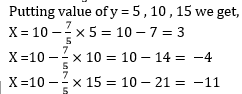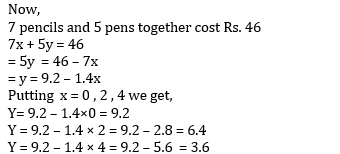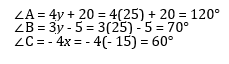FundooTutor

# EXERCISE 3.2

#### Let the cost of one pencil=Rs.X and Let the cost of one pen=Rs.Y According to the given conditions, we have: =5x + 7y = 50 =5x=50-7y =x=10-7/5yThree solutions of this equation can be written in a table as follows:

 x 3 -4 -11 y 5 10 15

####Three solutions of this equation can be written in a table as follows:

 x 0 2 4 y 9.2 6.4 3.6

# EXERCISE 3.7

#### By using equation (i), we obtain x – y = – 40 -15 – y = – 40 y = -15 + 40 = 25